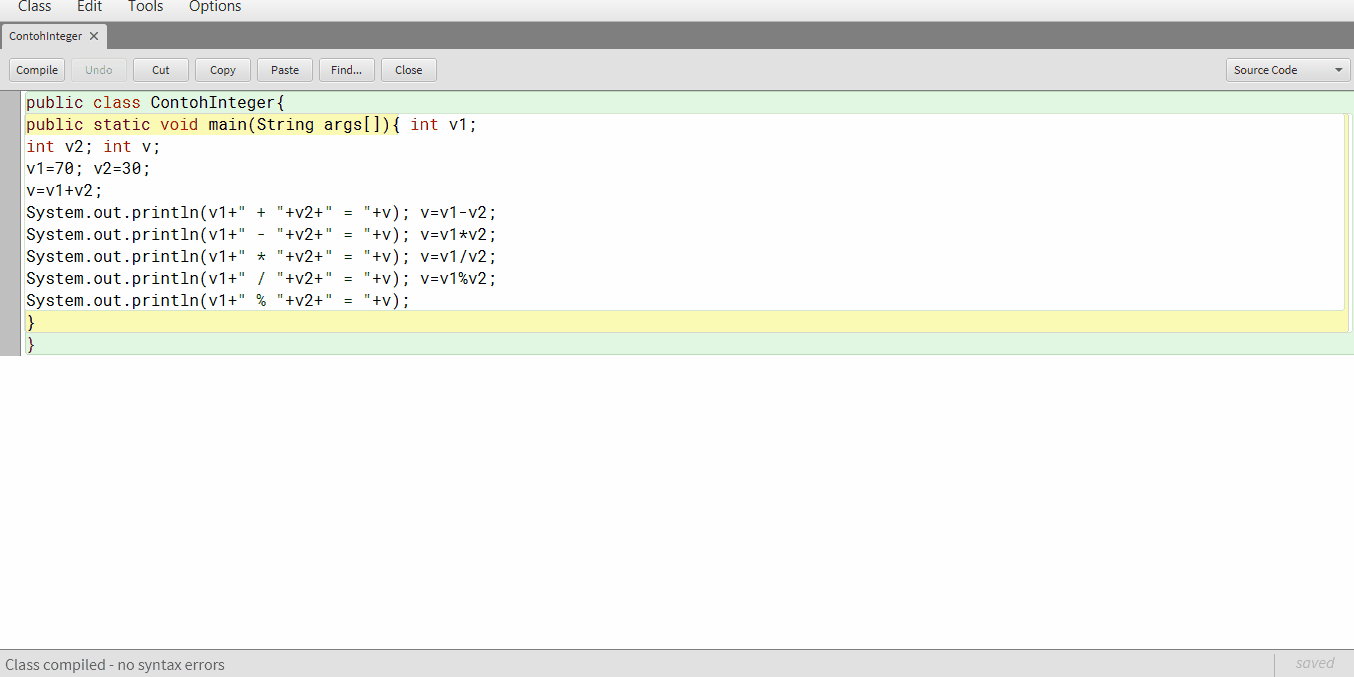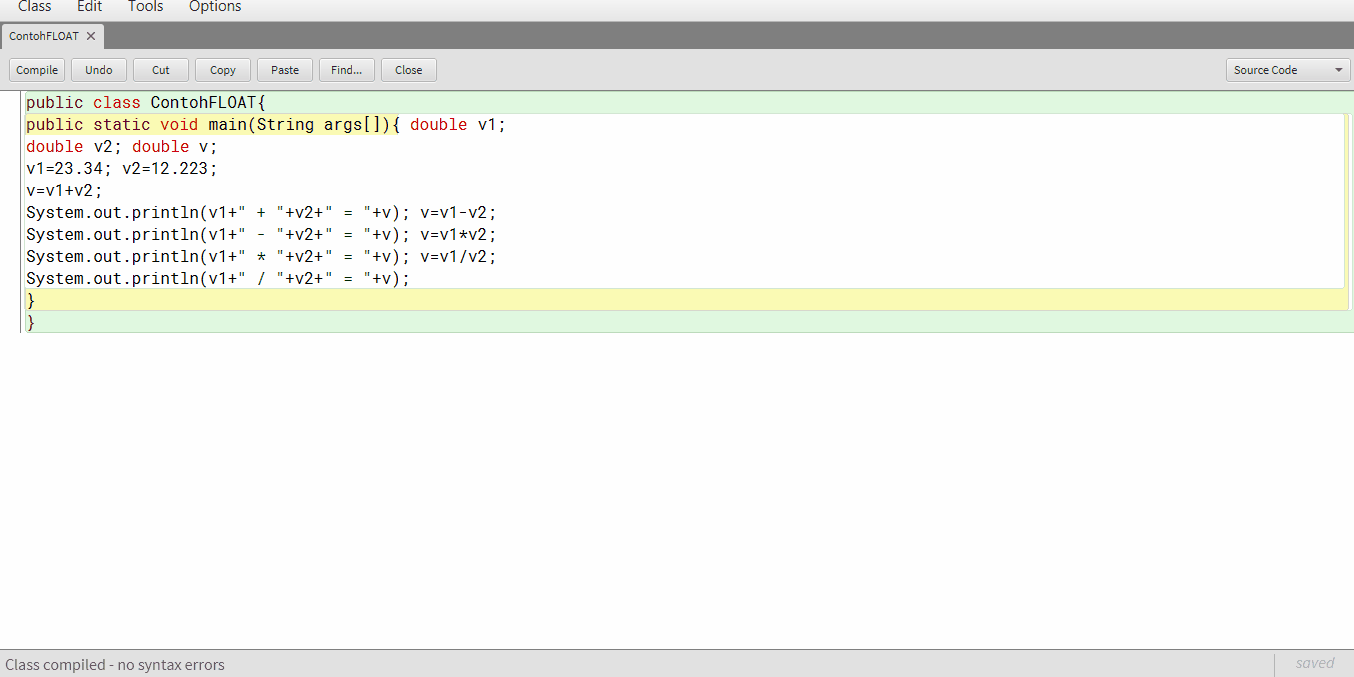# Use of variable JAVA

### Use of variable

Variable is a memory location that has been given a name and can be assigned a value during the execution of the program. Variable naming rules together with other programming languages ​​such as C, C ++, Pascal, etc.
Java coding examples using an integer data type variables:
 01 public class ContohInteger{
 02 public static void main(String args[]){ int v1;
 03 int v2; int v;
 04 v1=70; v2=30;
 05 v=v1+v2;
 06 System.out.println(v1+” + “+v2+” = “+v); v=v1-v2;
 07 System.out.println(v1+” – “+v2+” = “+v); v=v1*v2;
 08 System.out.println(v1+” * “+v2+” = “+v); v=v1/v2;
 09 System.out.println(v1+” / “+v2+” = “+v); v=v1%v2;
 10 System.out.println(v1+” % “+v2+” = “+v);
 11 }
 12 }coding results, will result in an integer (integer) of the accretion process, subtraction, division, multiplication.
Examples of java coding using variable data type float or double:
 01 public class Contoh3{
 02 public static void main(String args[]){ double v1;
 03 double v2; double v;
 04 v1=23.34; v2=12.223;
 05 v=v1+v2;
 06 System.out.println(v1+” + “+v2+” = “+v); v=v1-v2;
 07 System.out.println(v1+” – “+v2+” = “+v); v=v1*v2;
 08 System.out.println(v1+” * “+v2+” = “+v); v=v1/v2;
 09 System.out.println(v1+” / “+v2+” = “+v);
 10 }
 11 }coding results, will produce a number with fractional numbers (float / double) of the accretion process, subtraction, division, multiplication.